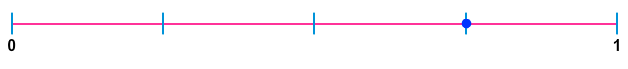# Fractions on Number Lines

Rate 0 stars Common Core
Quiz size:
Message preview:
Someone you know has shared quiz with you:

To play this quiz, click on the link below:

https://www.turtlediary.com/quiz/fractions-on-number-lines.html

Hope you have a good experience with this site and recommend to your friends too.

Login to rate activities and track progress.
Login to rate activities and track progress.

Consider the number line:Let's identify the fraction represented by the dot on the number line.

To identify the fraction, count the number of segments between 0 and 1.There are 4 segments.

Now, count the number of segments between 0 and the given dot.There are 3 segments.

So, the fraction represented on the given number line is
 3 4
.

ds

A B C D E F G H I J K L M N O P Q R S T U V W X Y Z

### Help

##### Remember :

The smallest number is the one that comes first while counting.

##### Solution :

To arrange the given numbers in order from smallest to greatest, find the smallest number among all the given numbers.

21,27,23

21 is the smallest number.